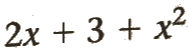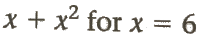## Labels

### GED Math Review: Evaluating Polynomial Expressions

Questions:

A. Evaluate the expression for x = 3, 2, 1, and 0.

1. 13 + 6x
2.B. evaluate the expression for the given values of the variables.
3. w + 6 for w = 11
4. r - 9 for r = 20
5. 17 - 2c for c = 7
6.7.8.9. m + 2m + 3 for m = 12
10. 9a - 5a for a = 7
11. 4 x (21 - 3h) for h = 5
12. 7m - 9n for m = 7 and n = 5
13.14. 3x + 4y ÷ 2 for x = 7 and y = 10
15. The formula P = 2l + 2w gives the perimeter P of a rectangular room with length
l and width w. A rectangular living room is 26 feet long and 21 feet wide. What is the perimeter of the room?
16. The formula c = 5(f - 21) ÷ 9 gives the Celsius temperature in c degrees for a Fahrenheit temperature of f degrees. What is the Celsius temperature for a Fahrenheit temperature of 122 degrees?

1. 31, 25, 19, and 13
2. 18, 11, 6, and 3
3. 17
4. 11
5. 3
6. 21
7. 4
8. 42
9. 39
10. 28
11. 24
12. 4
13. 22
14. 41
15. 94
16. 50 celsius

Reference:

Complete Test Preparation for the GED Test 2014 by Steck-Vaughn